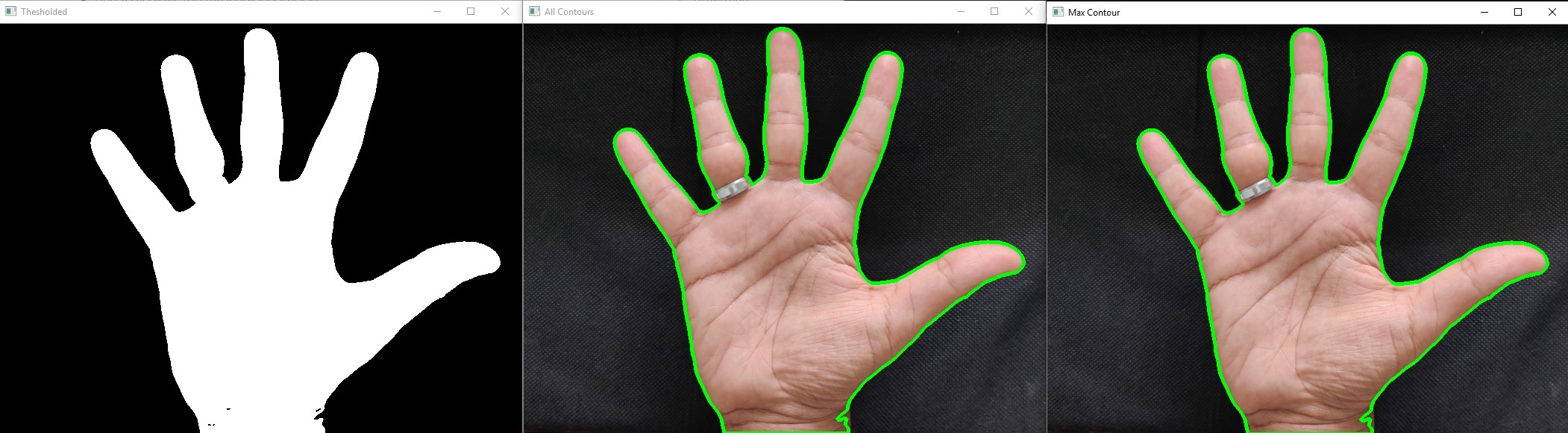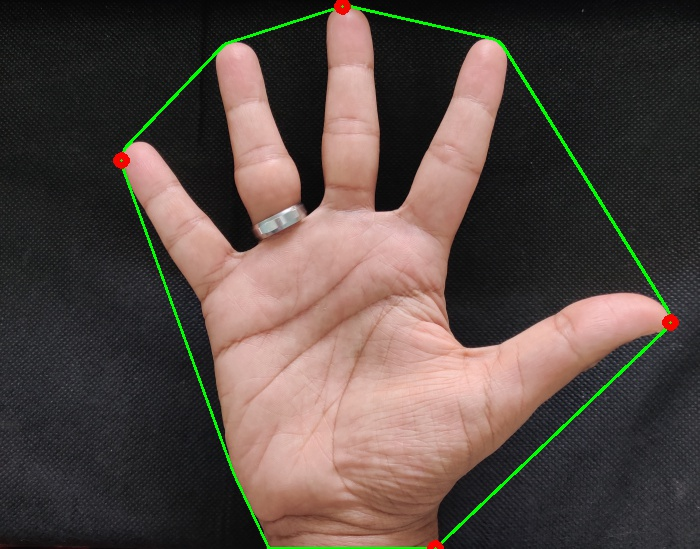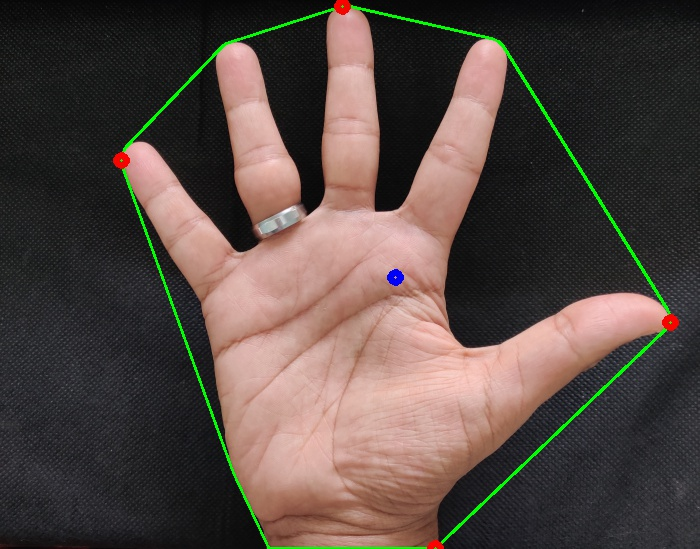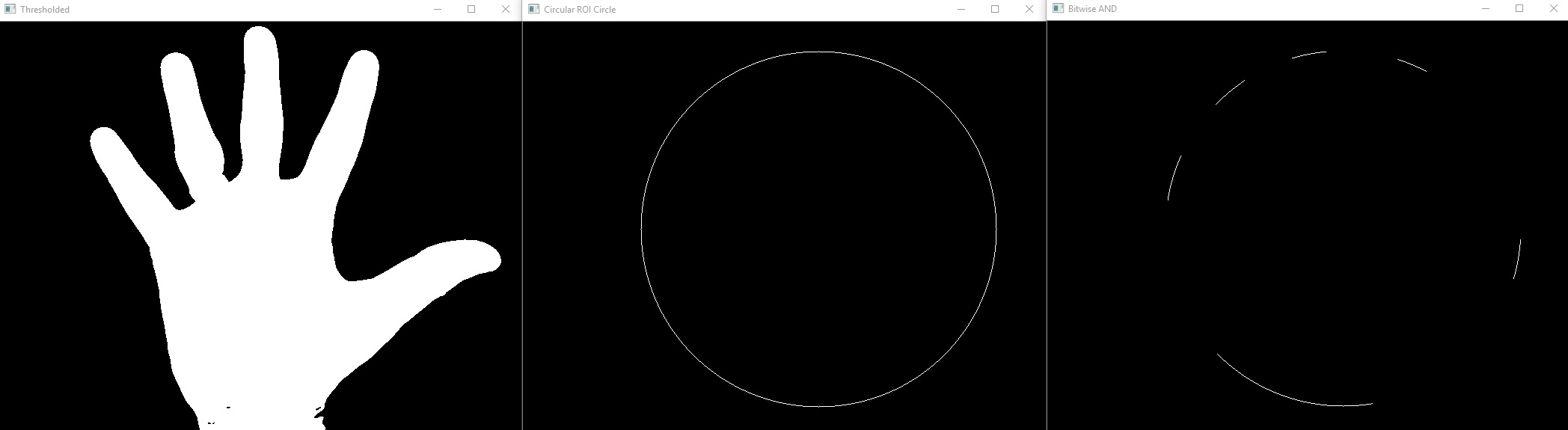# Hand Gesture Recognition using Python and OpenCV - Part 2

Computer Vision | 25 April 2017

This is a follow-up post of my tutorial on Hand Gesture Recognition using OpenCV and Python. Please read the first part of the tutorial here and then come back.

In the previous tutorial, we have used Background Subtraction, Motion Detection and Thresholding to segment our hand region from a live video sequence. In this tutorial, we will take one step further to recognize the fingers as well as predict the number of fingers (count) in the live video sequence.

Note: This tutorial assumes that you have knowledge in using OpenCV, Python, NumPy and some basics of Computer Vision and Image Processing. If you need to setup environment on your system, please follow the instructions posted here and here.

### Count My Fingers

Having segmented the hand region from the live video sequence, we will make our system to count the fingers that are shown via a camera/webcam. We cannot use any template (provided by OpenCV) that is available to perform this, as it is indeed a challenging problem.

The entire code from my previous tutorial (Hand Gesture Recognition-Part 1) can be seen here for reference. Note that, we have used the concept of Background Subtraction, Motion Detection and Thresholding to segment the hand region from a live video sequence.

We have obtained the segmented hand region by assuming it as the largest contour (i.e. contour with the maximum area) in the frame. If you bring in some large object inside this frame which is larger than your hand, then this algorithm fails. So, you must make sure that your hand occupies the majority of the region in the frame.

We will use the segmented hand region which was obtained in the variable hand. Remember, this hand variable is a tuple having thresholded (thresholded image) and segmented (segmented hand region). We are going to utilize these two variables to count the fingers shown. How are we going to do that?

There are various approaches that could be used to count the fingers, but we are going to see one such approach in this tutorial. This is a faster approach to perform hand gesture recognition as proposed by Malima et.al. The methodology to count the fingers (as proposed by Malima et.al) is shown in the figure below.

As you can see from the above image, there are four intermediate steps to count the fingers, given a segmented hand region. All these steps are shown with a corresponding output image (shown in the left) which we get, after performing that particular step.

### Four Intermediate Steps

1. Find the convex hull of the segmented hand region (which is a contour) and compute the most extreme points in the convex hull (Extreme Top, Extreme Bottom, Extreme Left, Extreme Right).
2. Find the center of palm using these extremes points in the convex hull.
3. Using the palm’s center, construct a circle with the maximum Euclidean Distance (between the palm’s center and the extreme points) as radius.
4. Perform bitwise AND operation between the thresholded hand image (frame) and the circular ROI (mask). This reveals the finger slices, which could further be used to calcualate the number of fingers shown.

Below you could see the entire function used to perform the above four steps.

• Input - thresholded (thresholded image) and segmented (segmented hand region or contour)
• Output - count (Number of fingers).

### recognize.pycode

1
2
3
4
5
6
7
8
9
10
11
12
13
14
15
16
17
18
19
20
21
22
23
24
25
26
27
28
29
30
31
32
33
34
35
36
37
38
39
40
41
42
43
44
45
46
47
48
49
50
51
52
53
54
55
56
57
58
#--------------------------------------------------------------
# To count the number of fingers in the segmented hand region
#--------------------------------------------------------------
def count(thresholded, segmented):
# find the convex hull of the segmented hand region
chull = cv2.convexHull(segmented)

# find the most extreme points in the convex hull
extreme_top    = tuple(chull[chull[:, :, 1].argmin()])
extreme_bottom = tuple(chull[chull[:, :, 1].argmax()])
extreme_left   = tuple(chull[chull[:, :, 0].argmin()])
extreme_right  = tuple(chull[chull[:, :, 0].argmax()])

# find the center of the palm
cX = int((extreme_left + extreme_right) / 2)
cY = int((extreme_top + extreme_bottom) / 2)

# find the maximum euclidean distance between the center of the palm
# and the most extreme points of the convex hull
distance = pairwise.euclidean_distances([(cX, cY)], Y=[extreme_left, extreme_right, extreme_top, extreme_bottom])
maximum_distance = distance[distance.argmax()]

# calculate the radius of the circle with 80% of the max euclidean distance obtained

# find the circumference of the circle
circumference = (2 * np.pi * radius)

# take out the circular region of interest which has
# the palm and the fingers
circular_roi = np.zeros(thresholded.shape[:2], dtype="uint8")

# draw the circular ROI
cv2.circle(circular_roi, (cX, cY), radius, 255, 1)

# take bit-wise AND between thresholded hand using the circular ROI as the mask
# which gives the cuts obtained using mask on the thresholded hand image

# compute the contours in the circular ROI
(_, cnts, _) = cv2.findContours(circular_roi.copy(), cv2.RETR_EXTERNAL, cv2.CHAIN_APPROX_NONE)

# initalize the finger count
count = 0

# loop through the contours found
for c in cnts:
# compute the bounding box of the contour
(x, y, w, h) = cv2.boundingRect(c)

# increment the count of fingers only if -
# 1. The contour region is not the wrist (bottom area)
# 2. The number of points along the contour does not exceed
#     25% of the circumference of the circular ROI
if ((cY + (cY * 0.25)) > (y + h)) and ((circumference * 0.25) > c.shape):
count += 1

return count


Each of the intermediate step requires some understanding of image processing fundamentals such as Contours, Bitwise-AND, Euclidean Distance and Convex Hull.

### Contours

The outline or the boundary of the object of interest. A contour is defined as the line that joins all the points along the boundary of an image that have the same intensity. cv2.findContours() function in OpenCV help us find contours in a binary image. If you pass a binary image to this function, it returns a list of all the contours in the image. Each of the elements in this list is a numpy array that represents the (x, y) coordinate of the boundary points of the contour (or the object).

### Bitwise-AND

Performs bit-wise logical AND between two objects. You could visually think of this as using a mask and extracting the regions in an image that lie under this mask alone. OpenCV provides cv2.bitwise_and() function to perform this operation - Bitwise AND.

### Euclidean Distance

This is the distance between two points given by the equation shown here. Scikit-learn provides a function called pairwise.euclidean_distances() to calculate the Euclidean distance from one point to multiple points in a single line of code - Pairwise Euclidean Distance. After that, we take the maximum of all these distances using NumPy’s argmax() function.

### Convex Hull

You can think of convex hull as a dynamic, stretchable envelope that wraps around the object of interest. To read more about it, please visit this link.

Update: I thought of adding some more explanation to the algorithm and code. Let's understand this algorithm and code with an image (one frame) so that each step is clear for us.

### Segmenting the hand

Let’s look at the following code snippet which is a python function that segments the hand region from the image (single frame).

### recognize-image.pycode

1
2
3
4
5
6
7
8
9
10
11
12
13
14
15
16
17
18
19
20
21
22
23
24
25
26
27
28
29
30
#---------------------------------------------
# To segment the region of hand in the image
#---------------------------------------------
def segment(image, grayimage, threshold=75):
# threshold the image to get the foreground which is the hand
thresholded = cv2.threshold(grayimage, threshold, 255, cv2.THRESH_BINARY)
print("Original image shape - " + str(image.shape))
print("Gray image shape - " + str(grayimage.shape))

# show the thresholded image
cv2.imshow("Thesholded", thresholded)

# get the contours in the thresholded image
(_, cnts, _) = cv2.findContours(thresholded.copy(), cv2.RETR_EXTERNAL, cv2.CHAIN_APPROX_SIMPLE)

# return None, if no contours detected
if len(cnts) == 0:
return
else:
# analyze the contours
print("Number of Contours found = " + str(len(cnts)))
cv2.drawContours(image, cnts, -1, (0, 255, 0), 3)
cv2.imshow('All Contours', image)

# based on contour area, get the maximum contour which is the hand
segmented = max(cnts, key=cv2.contourArea)
cv2.drawContours(image, segmented, -1, (0, 255, 0), 3)
cv2.imshow('Max Contour', image)

return (thresholded, segmented)

• Line 4 initializes the function with input color image image, input grayscale image grayimage and a threshold variable that we can tune based on the lighting conditions of our image or video.
• Line 6 thresholds the grayscale image with respect to the threshold that we have choosen. Anything above the threshold is transformed to white and anything below the threshold is transformed to black. You can read more on how to choose the threshold or what thresholding you should use here.
• Line 7 and 8 prints the original color image and input gray image shapes for our reference.
• Line 11 displays the thresholded image.
• Line 14 is where the magic happens. We pass the thresholded image thresholded to OpenCV’s cv2.findContours() function with some additional parameters that the function expects. This returns all the contours in the thresholded image. Notice how we unpack the return values.
• cnts is a list of all the contours in the thresholded image. Each element in this list is a numpy array that represents the (x, y) coordinate of the boundary points of the contour (or the object).
• Line 17 checks the length of contours found, and if its 0, it simply returns nothing.
• Line 21 prints the number of contours found.
• Line 22 draws all the contours found in the thresholded image using OpenCV’s cv2.drawContours() function.
• Line 23 displays all the contours present in the image.
• Line 26 finds the maximum contour in the image based on key cv2.contourArea. Here, we assume that our hand has the largest area in the image. If some object larger than our hand is found in the image, we need to handle this line of code.
• Line 27 draws the contour with maximum area which in our case is the hand.
• Line 28 displays the contour with maximum area in the image.
• Line 30 returns a tuple that has thresholded image and segmented contour (which is the hand).Figure 2. Thresholding and Segmenting Hand region from an image (a single frame). (a) Thresholded image (b) All Contours (c) Max Contour

### Finding the count of fingers

Let’s look at the following code snippets which is inside a python function that is used to count the number of fingers in the image (single frame).

I have splitted the code snippet into smaller pieces so that we can understand this line by line. Make sure to check the complete function here.

Firstly, we pass the segmented contour which is a numpy array that contains all the boundary points (x,y) coordinates. To understand this, let’s print the type and shape of the segmented contour with first 5 boundary points inside it.

### recognize-image.pycode

1
2
3
print("Type of Contour: " + str(type(segmented)))
print("Contour shape: " + str(segmented.shape))
print("First 5 points in contour: " + str(segmented[:5]))

1
2
3
4
5
6
7
8
9
10
Type of Contour: <class 'numpy.ndarray'>
Contour shape: (1196, 1, 2)
First 5 points in contour:
[
[[342   6]]
[[341   7]]
[[338   7]]
[[337   8]]
[[336   8]]
]


The next step is to find the extreme points in this contour. How? Here is another magic function in OpenCV - cv2.convexHull() which returns the farthest boundary points of a contour. To understand it clearly, let’s pass in our segmented contour to cv2.convexHull() function.

### recognize-image.pycode

1
2
3
4
5
6
7
8
9
# find the convex hull of the segmented hand region
chull = cv2.convexHull(segmented)

print("Type of Convex hull: " + str(type(chull)))
print("Length of Convex hull: " + str(len(chull)))
print("Shape of Convex hull: " + str(chull.shape))

cv2.drawContours(image, [chull], -1, (0, 255, 0), 2)
cv2.imshow("Convex Hull", image)

1
2
3
Type of Convex hull: <class 'numpy.ndarray'>
Length of Convex hull: 30
Shape of Convex hull: (30, 1, 2)


For those of you new to python and numpy array, you can write a single line of code to find the extreme points after getting the convex hull. Concept of argmin() and argmax() is used to achieve this as shown below.

### recognize-image.pycode

1
2
3
4
5
print(chull[:,:,1])
print(chull[:,:,1].argmin())
print(chull[chull[:,:,1].argmin()])
print(chull[chull[:,:,1].argmin()])
print(tuple(chull[chull[:,:,1].argmin()]))

1
2
3
Type of Convex hull: <class 'numpy.ndarray'>
Length of Convex hull: 30
Shape of Convex hull: (30, 1, 2)


Above code sample is used to find extreme right boundary point in the convex hull. To find the extreme right boundary point,

• We choose the x-axis column of the convex hull using chull[:, :, 0] where 0 indicates the first column.
• We then find the index of maximum number in x-axis column using chull[:, :, 0].argmax().
• We then use that max index to grab the boundary point (x, y) using chull[chull[:,:,1].argmin()].
• We then get the first indexed element and convert it to a tuple so that it doesn’t get changed as tuples are immutable using tuple(chull[chull[:,:,1].argmin()])

Similar one line of code is used to find all the extreme points of the convex hull as shown below.

### recognize-image.pycode

1
2
3
4
5
6
7
8
9
10
11
12
13
14
15
16
17
# find the most extreme points in the convex hull
extreme_top    = tuple(chull[chull[:, :, 1].argmin()])
extreme_bottom = tuple(chull[chull[:, :, 1].argmax()])
extreme_left   = tuple(chull[chull[:, :, 0].argmin()])
extreme_right  = tuple(chull[chull[:, :, 0].argmax()])

print("Extreme Top : " + str(extreme_top))
print("Extreme Bottom : " + str(extreme_bottom))
print("Extreme Left : " + str(extreme_left))
print("Extreme Right : " + str(extreme_right))

cv2.drawContours(image, [chull], -1, (0, 255, 0), 2)
cv2.imshow("Extreme Points in Convex Hull", image)

1
2
3
4
Extreme Top    : (342, 6)
Extreme Bottom : (435, 548)
Extreme Left   : (121, 160)
Extreme Right  : (670, 322)Figure 4. Extreme points in Convex Hull of the segmented image

### recognize-image.pycode

1
2
3
4
5
6
7
8
9
10
11
12
# find the center of the palm
cX = int((extreme_left + extreme_right) / 2)
cY = int((extreme_top + extreme_bottom) / 2)
print("Center point : " + str(tuple((cX,cY))))

cv2.drawContours(image, [chull], -1, (0, 255, 0), 2)
cv2.circle(image, (cX, cY), radius=5, color=(255,0,0), thickness=5)
cv2.imshow("Extreme Points in Convex Hull", image)

1
Center point : (395, 277)Figure 5. Center point with Extreme points in Convex Hull of the segmented image

Once we have the center point and extreme points, we need to find the euclidean distance from the center point to each of the extreme point. We do that in a single line of code using scikit-learn’s pairwise.euclidean_distances(). After this, we find the maximum distance with respect to each of the four euclidean distances and form a circumference with radius as 80% of the maximum distance.

### recognize-image.pycode

1
2
3
4
5
6
7
8
9
10
11
12
13
14
15
# find the maximum euclidean distance between the center of the palm
# and the most extreme points of the convex hull
distances = pairwise.euclidean_distances([(cX, cY)], Y=[extreme_left, extreme_right, extreme_top, extreme_bottom])
max_distance = distances[distances.argmax()]

# calculate the radius of the circle with 80% of the max euclidean distance obtained

# find the circumference of the circle
circumference = (2 * np.pi * radius)

print("Euclidean Distances : " + str(distances))
print("Max Euclidean Distance : " + str(max_distance))
print("Circumference : " + str(circumference))

1
2
3
4
Euclidean Distance : [297.93455657 278.65749586 276.13402543 273.93612394]
Max Euclidean Distance : 297.9345565724124
Circumference : 1495.3981031087415


Once we have the center of the convex hull, radius and circumference using the maximum distance, we then construct a numpy array filled with zeros called circular_roi using the thresholded image’s shape. We then draw a circle in this circular_roi with the center of convex hull as the center and radius calculated above.

Next magic in our pipeline is OpenCV’s cv2.bitwise_and() function which is a bitwise operation that you can read more about it here.

In simple terms, you have an image and a mask, and pass these to cv2.bitwise_and() function, the output will be the region where both image and mask had same intensity values. In our case, it displays the cuts in the fingers because we have used thresholded image and circular_roi as the mask.

### recognize-image.pycode

1
2
3
4
5
6
7
8
9
10
11
12
13
# initialize circular_roi with same shape as thresholded image
circular_roi = np.zeros(thresholded.shape[:2], dtype="uint8")
print("Circular ROI shape : " + str(circular_roi.shape))
cv2.imshow("Thresholded", thresholded)

# draw the circular ROI with radius and center point of convex hull calculated above
cv2.circle(circular_roi, (cX, cY), radius, 255, 1)
cv2.imshow("Circular ROI Circle", circular_roi)

# take bit-wise AND between thresholded hand using the circular ROI as the mask
# which gives the cuts obtained using mask on the thresholded hand image
cv2.imshow("Bitwise AND", circular_roi)Figure 6. Construct a circle with center point and radius obtained from convex hull and do bit-wise AND with thresholded image

Now, we have a circular_roi which has the finger cuts obtained from the thresholded image. To calculate the count of the fingers, we need to exclude the wrist portion in the circular_roi so that we focus only on the fingers.

To achieve this, we make use of cv2.findContours() again to find all the contours in circular_roi.

To remove the wrist, we can sort contours based on area and leave the first contour (as it will be the wrist).

### recognize-image.pycode

1
2
3
4
5
# compute the contours in the circular ROI
(_, cnts, _) = cv2.findContours(circular_roi.copy(), cv2.RETR_EXTERNAL, cv2.CHAIN_APPROX_NONE)
print("Number of Contours found = " + str(len(cnts)))
cv2.drawContours(image, cnts, -1, (0, 255, 0), 3)
cv2.imwrite("resources/count-contours.jpg", image)


Once we have the contours after bitwise-and done on circular_roi, we can easily find the count of fingers by two approaches.

#### Approach 1

By sorting the contours based on area, the first contour will obviously be the wrist. So, we can ignore it and find the length of the remaining contours which will be the count of fingers shown in the image.

### recognize-image.pycode

1
2
cntsSorted = sorted(cnts, key=lambda x: cv2.contourArea(x))
print("Count of fingers : " + str(len(cntsSorted[1:])))

1
Count of fingers : 5


#### Approach 2

For each of the contour, we can find the bounding rectangle of the contour using cv2.boundingRect() function. This returns 4 numbers where (x,y) is the top-left coordinate of the rectangle and (w,h) is the width and height of the rectangle.

Check 1: To filter out the wrist area, we can use vertical (y) axis to check whether y + h of a contour is lesser than center point cY of the convex hull. Sometimes, the thumb of our hand is flexible i.e it can move horizontally or vertically. To compensate for this, we marginally increase the cY of the convex hull by a factor 0.25 i.e. we use cY + (cY * 0.25) to check against y + h. You can still tune this value based on your use case. For me, 0.25 was good to go!

Check 2: To filter out the wrist area even more, we could also use the perimeter of the circle (circumference). When we loop over the contour, we find that a single contour is a numpy array. Inside this numpy array, we have the boundary points that forms the contour. We can check whether the number of boundary points of a contour c.shape lie within a limit. Again, I have chosen this limit as circumference * 0.25. You can still tune this value based on your use case. For me, 0.25 was good to go!

### recognize-image.pycode

1
2
3
4
5
6
7
8
9
10
11
12
13
14
15
16
17
18
19
20
count = 0

# loop through the contours found
for c in cnts:
print(type(c))
# compute the bounding box of the contour
(x, y, w, h) = cv2.boundingRect(c)

cv2.rectangle(image, (x,y), (x+w, y+h), (0,255,0), 2)
cv2.putText(image,'C' + str(i), (x,y), cv2.FONT_HERSHEY_SIMPLEX, 1,(255,255,255), 4)

# increment the count of fingers only if -
# 1. The contour region is not the wrist (bottom area)
# 2. The number of points along the contour does not exceed
#     25% of the circumference of the circular ROI
if ((cY + (cY * 0.25)) > (y + h)) and ((circumference * 0.25) > c.shape):
count += 1

print("Count of fingers : " + str(len(cntsSorted[1:])))
cv2.imshow("Contours of fingers with box", image)

1
Count of fingers : 5


Figure 8 has all the intermediate steps that we have performed to build this pipeline. I have marked each of the intermediate step in different colors for clear understanding.

### Results

You can download the entire code to perfom Hand Gesture Recognition here. Clone this repository using

### Commandshell

1
git clone https://github.com/Gogul09/gesture-recognition.git


in a Terminal/Command prompt. Then, get into the folder and type

### Commandshell

1
python recognize.py


Note: Do not shake your webcam during the calibration period of 30 frames. If shaken during the first 30 frames, the entire algorithm will not perform as we expect.

After that, you can bring in your hand into the bounding box, show gestures and the count of fingers will be displayed accordingly. I have included a demo of the entire pipeline below.